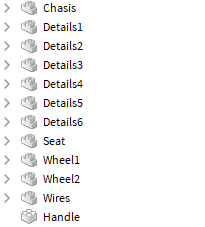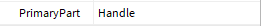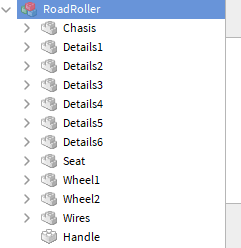# Model not rotating

Hello, so I’ve welded a model to my Character using a WeldConstraint, and want to rotate it after it’s been welded using:

``````Model:SetPrimaryPartCFrame(PrimaryPart.CFrame * CFrame.Angles(math.rad(XAngle)), 0, 0)
``````

But, after the code has been ran, the rotation of the model doesn’t change at all, I’ve tried running the code on the model previous to welding it and it works, I doubt it’s :SetPrimaryPartCFrame(), does anybody know a way to keep the model welded to the Character, but letting me also tween or change it’s rotation afterwards?

I’ve tried using:

``````Model.PrimaryPart.CFrame = Model.PrimaryPart.CFrame * CFrame.Angles(math.rad(XAngle), 0, 0)
``````

Expecting a different result, but it still didn’t change, What should I do?

Is PrimaryPart an actual object of your model if not then try putting in your script the PrimaryPart of the actual object u have put as PrimaryPart for the model. If u can try showing us all the part there is in your model

Yes, PrimaryPart is set in the model, the code runs fine, but the rotation doesn’t change. And the output does not show any types of errors.

I mean that is PrimaryPart a part that is actually the children of the model?yep

It’s all grouped in the model, sorry I didn’t show it.Then u should try this

`` Model:SetPrimaryPartCFrame(Model.Handle.CFrame * CFrame.Angles(math.rad(XAngle)))``

I appreciate the help but, it didn’t work

1 Like

I think i found the problem

`````` Model:SetPrimaryPartCFrame(Model.Handle.CFrame * CFrame.Angles(math.rad(XAngle),0,0))
``````

U need to add some 0 so that it look like this x,y,z or else it will overide

i did do that, i just truncated the code so it wasn’t as long, sorry for the misunderstanding

1 Like

Ok, I fixed it, instead of using a WeldConstraint, I used a ManualWeld in this shown way:

``````local Weld = Instance.new("ManualWeld")
Weld.Part0 = Roller.PrimaryPart
Weld.Part1 = Character["Right Arm"]
Weld.C0 = Roller.PrimaryPart.CFrame:inverse()
Weld.C1 = Character["Right Arm"].CFrame:inverse()
Weld.Parent = Weld.Part0

wait(1)

Weld.C0 = (Roller.PrimaryPart.CFrame * CFrame.Angles(math.rad(XAngle), 0, 0)):inverse()
Weld.C1 = Character["Right Arm"].CFrame:inverse()
``````

Don’t know if I can tween it yet but I’ll try

1 Like

That’s cool u fix it yourself btw here is a link on how to tween models Introduction to Tweening Models